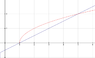# Double integral and limits

santais

## Homework Statement

Calculate the integral:

$∫ ∫_R (x+2y)^{-(1/2)} dxdy$

Where R is defined as points (x,y) which satisfy:

x-2y ≤ 1 and x ≥ y2 + 1

## Homework Equations

So basically I'm completely stuck on this exerzice. As far as I can see, you could make the x limit go from y2 +1 to infinity. However, looking at what I have to integrate, and the possible solutions, it doesn't seem to be a possible answer.

Also the y limits seems quite unclear how to determine.

I hope that some of you out there could give me a little push, to get in the right direction with this assigment :)

Gold Member
Hi santais

You should always plot the graph to understand the region R, defined by the two given equations. See the attached graph.

Now that you can see the required region of integration, describe it, so you can define the limits with respect to x and y:
For ##y## fixed, ##x## varies from ##x = y^2 + 1## to ##x = 2y +1##
y varies from y = 0 to y = 2

#### Attachments

•region.png
3.4 KB · Views: 404
Last edited:
tt2348
Try drawing out the region defined, showing the area you're integrating over first. Also integrate x as a function of y for your limits

santais
So the limits would be somehow:

1 <= x <= 5 and (x-1)/2 <= y <= Sqrt[x-1]

Homework Helper
Why "somehow"? Your constraints, $x- 2y\le 1$ which is equivalent to $y\ge (x-1)/2$, and $x\ge y^2+ 1$, mean that the region of integration lies between those two graphs as shown by sharks. They intersect when $x= 2y+ 1= y^2+ 1$ which reduces to $y^2- 2y= y(y- 2)= 0$ so y= 0 or y= 2. When y= 0, x= 2(0)+ 1= 1 and when y= 2, x= 2(2)+ 1= 5. The points of intersection of x= 2y+ 1 and $x= y^2+ 1$ are (1, 0) and (5, 2). To cover that we can take x ranging from 0 to 5. For each x, y ranges from the line, y= (x- 1)/2, to the parabola, $y= \sqrt{x- 1}$ (positive branch since, from the graph, we want the point to be above the x-axis).
$$\int_{x=1}^5\int_{y= (x-1)/2}^\sqrt{x-1} f(x,y)dydx$$

But, especially because we are given the parabola in $y^2$, we could also do the integration with respect to y second. y ranges from 0 to 2 and, for each y, x ranges from the parabola, x= y^2+ 1, on the left, to the line, x= 2y+ 1, on the right. That is, we could also do the integral as
$$\int_{y= 0}^2\int_{x=y^2+1}^{2y+1} f(x,y) dxdy$$

santais
ARh okay. It makes so much more sense now :) And thanks for the fast replies.

Solved the assignment now. At least it corresponds to the answer I get in Mathematica, so I'll stick with this answer :)

Thanks for the replies!